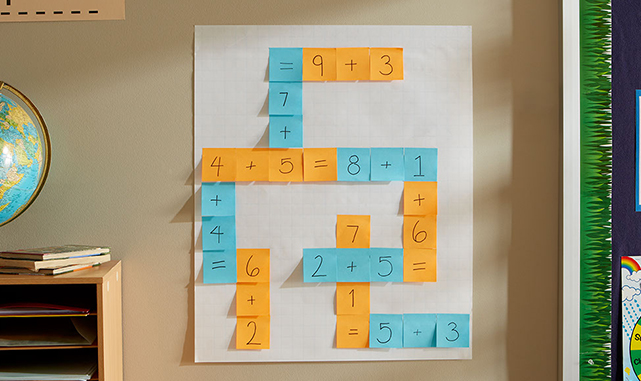# Criss Cross Equivalents

Lesson Plan, Grades 1-3, Math, Post-it, School Smart### Description

Use Post-it® Notes to teach math equivalents.

### Supplies Needed

Post-it Original Notepad, 3 X 3 in
School Smart Non-Toxic Quick Dry Washable Marker

## Instructions

### 1

Divide the students into pairs. Give each team 5 minutes to create as many addition equations as they can.

### 2

To get started, one student writes an unsolved addition equation on Post-it® Super Sticky Notes with each piece of the equation on separate note.

### 3

His or her teammate then writes an equation adding up to the sum. Since the equations build off of the previous one, the notes take on a crossword puzzle-like arrangement. Have your students continue writing down equations until time expires.

### 4

The pair of students that comes up with the most addition problems in the allotted time wins.

### 5

This math equation crossword can be used in subtraction, multiplication, division and fractions.